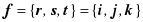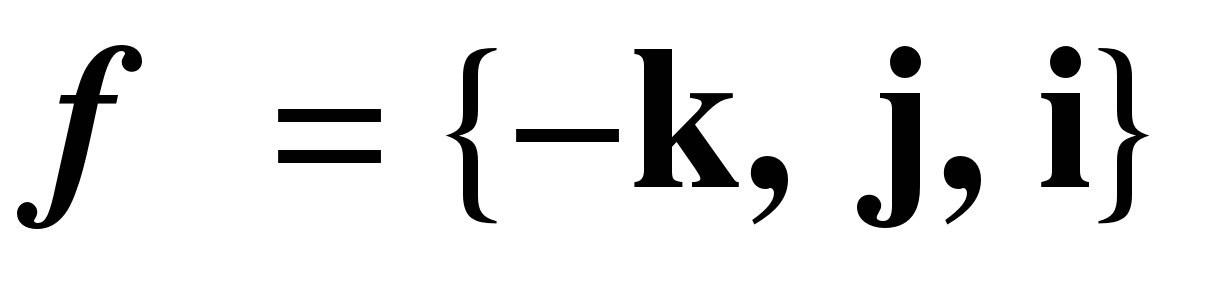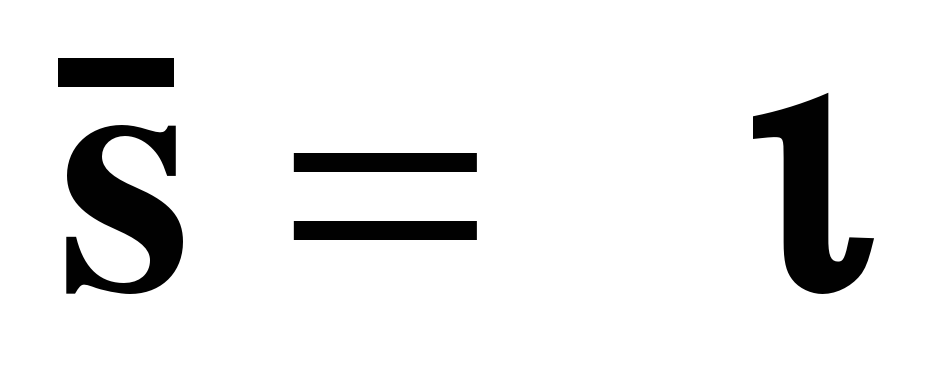# The Interdependence of Traveling Axes

 Page 4/14 Date 23.04.2018 Size 1.62 Mb. #45829

## The Interdependence of Traveling Axes

With the system in its initial state,, a rotation through an angular excursion of, about the transverse axis, would be expressed by -

We can check this by setting. Doing the calculation, we obtain. In words, the vertical axis is directed anteriorly, the sagittal axis is directed inferiorly, and the transverse axis is still transverse.

After the rotation of 90° about the vertical axis, the transverse axis was pointing posteriorly. Now, if we follow that rotation by a rotation about the transverse axis,, then the new orientation is given by -

Now, we apply the same process to the frame of reference, and then we can write out the expression in unbarred coordinates.

Note that the moving armature and the axis about which it rotates have been transformed by the same rotation about the vertical axis.

This simple situation leads to a fairly complex expression, but still an interpretable expression. If we introduce all the degrees of freedom in a gimbal joint, the expression of the solution rapidly passes beyond ready comprehension, therefore it is advisable to quickly move to computer calculators and models to deal with the kinematics of the joints.